ï»¿

### Introduzione

#### Abstract

En
We study a semi-linear initial-value problem arising from a Boltzmam like model of vahicular traffic an a motor way supposed infinite and without entrances and exits.We prove the existence and uniqueness of the local (in Time) "mild" solution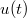$u(t)$ in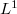$L^1$ and its positivity, if the initial condition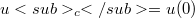$u_c = u(0)$ is positive and belougs to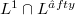$L^1 \cap L^âˆˆfty$. Finally,we consider the "mollified" version of the problem,already in another paper,and we show that unique strit solution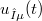$u_{Îµ}(t)$ is such that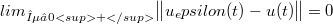$lim_{Îµ â†’ 0+} \big\Vert u_epsilon(t) - u(t) \big\Vert = 0$ uniformly in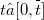$t âˆˆ [0, \buildrel \_ \over t]$ with$\buildrel \_ \over t$ suitably chosen.

DOI Code: §

Full Text: PDF